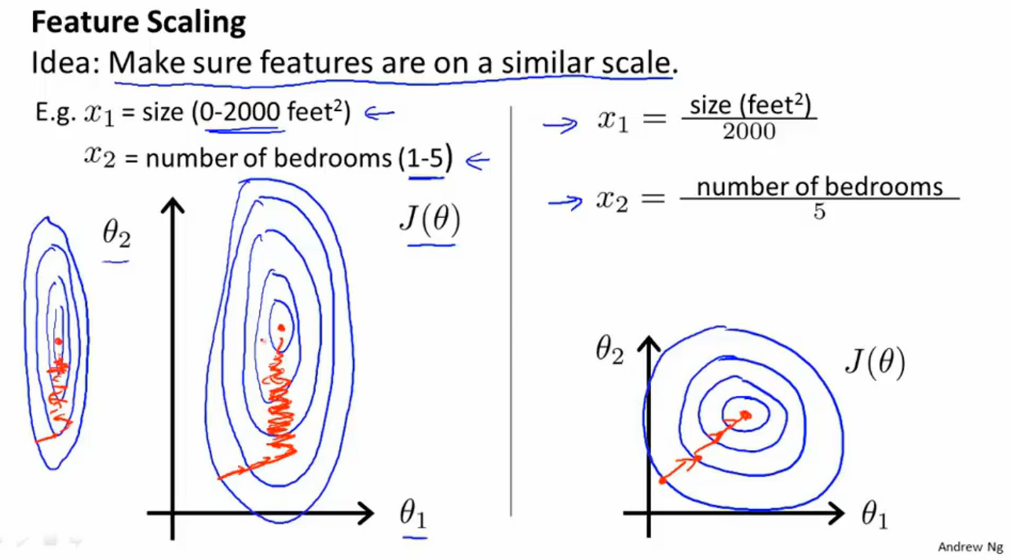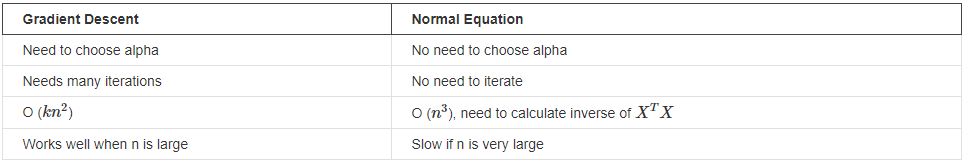ZhouYongyi's Personal Blog# 吴恩达《机器学习》课程学习笔记（三）—— 多元线性回归与多项式回归

## 一、多元线性回归（Multivariate Linear Regression）的数值计算法

### 1.多元假设方程（ The multivariable form of the hypothesis function）

$$x_3$$表示层数，​$$x_4$$​表示房屋的年龄，那么新的假设方程为：

$h_\theta=\theta_0+\theta_1x_1+\theta_2x_2+\theta_3x_3+\theta_4x_4$

$h_\theta=\theta_0+\theta_1x_1+\theta_2x_2+\theta_3x_3+…+\theta_nx_n \space\space\space\space\space\space(1.1)$

$h_\theta(x)=\left[\begin{array}{1} \theta_0 & \theta_1 & \theta_2 & … & \theta_n\end{array}\right] \left[\begin{array}{2} x_0\\x_1\\…\\x_n\end{array}\right]=\theta^Tx \space\space\space\space\space\space(1.2)$

$h_\theta(X)=X\theta \space\space\space\space\space\space(1.3)$

$$x_j^{(i)}=$$​第i个训练样例中第j个特征量的值

$$x^{(i)}=$$第i个训练样例的所有输入特征量组成的列向量

$$m=$$​训练样例的数量

$$n=|x^{(i)}|$$特征量的数量

### 2.代价函数（Cost function）

$J(\theta_1,\theta_2)=\frac{1}{2m}\sum_{i=1}^{m}(h_{\theta}(x^{(i)})-y^{(i)})^2 \space\space\space\space\space\space\space\space\space\space\space\space\space\space\space(1.4)$

$J(\theta)=\frac{1}{2m}（X\theta-Y）^T(X\theta-Y)\space\space\space\space\space\space\space\space\space\space\space\space\space\space\space(1.5)$

### 3.多元梯度下降算法（Gradient descent for multiple variables）

$\theta_{j}:=\theta_{j}-\alpha\frac{\partial }{\partial \theta_{j}}J(\theta_{0},\theta_{1}) \space\space\space\space\space\space\space\space\space\space\space\space\space\space\space(1.6)$

$\theta:=\theta-\alpha\bigtriangledown J(\theta)\space\space\space\space\space\space\space\space(1.7)$

$\frac{\partial J(\theta)}{\partial\theta_j}=\frac{1}{m}\sum_{i=1}^{m}(h_\theta(x^{(i)})-y^{(i)})\cdot x_j^{(i)} \space\space\space\space\space\space(1.9)$

$\frac{\partial J(\theta)}{\partial\theta_j}=\frac{1}{m}\overrightarrow{x_j}^T(X\theta-Y) \space\space\space\space\space\space(1.10)$

$\bigtriangledown J(\theta)=\frac{1}{m}X^T(X\theta-Y) \space\space\space\space\space\space(1.11)$

$\theta:=\theta-\frac{\alpha}{m}X^T(X\theta-Y) \space\space\space\space\space\space(1.12)$

### 4.特征量标准化（Feature normalization）

$0\leqslant x_1\leqslant 3$

$-2\leqslant x_2\leqslant 0.2$

$100000\leqslant x_3\leqslant 1000000000$

$0.00001\leqslant x_4\leqslant 0.0001$

• 数值计算是通过计算来迭代逼近的，如果特征量数量级相差太大，则很容易在运算过程中丢失。
• 直接把例子中的数据用在​$$h_\theta(x)=\theta_ix_i$$​上，那么整个方程的参数都是主要围绕$$x_3$$这个特征量来学习的。换个角度来理解：如果我们把其中​$$x_3$$​的单位由（单位量度）改为（100000个单位量度），那么的$$\theta_3x_3=10^5*\frac{x_3}{10^5}=\theta_{new} \space x_{new}$$即“天然地认为​$$x_new$$​的权重​$$\theta_{new}$$​很大”，这是不合理的。$x_i:=\frac{x_i-\mu}{max-min}\space\space\space\space\space\space(1.13)$

$x_i:=\frac{x_i-\mu_i}{s_i}\space\space\space\space\space\space(1.14)$

（注意，更多关于特征缩放的问题，请参考维基百科：Normalization_(statistics)，不同的取法对应了不同的标准化方法。)

### 5.学习率α（Learning rate）

$\theta_{j}:=\theta_{j}-\alpha\frac{\partial }{\partial \theta_{j}}J(\theta_{0},\theta_{1}) \space\space\space\space\space\space\space\space\space\space\space\space\space\space\space$

————————下面是私货————————————

1.在路由拥塞控制的时候，有这么一个流程：

## 二、多项式回归（Polynomial Regression）

### 1.多项式回归要注意的问题

$\theta_{j}:=\theta_{j}-\alpha\frac{\partial }{\partial \theta_{j}}J(\theta) \space\space\space\space\space\space\space\space\space\space\space\space\space\space\space(2.1)$

### 2.数值计算法求回归参数θ与解方程组的关系

• 两者都是通过迭代运算，最终收敛。
• 若方程组有解，那么数值解的精度δ可以自定，比如设置两次迭代所得根的差值δ < 0.0001时停止迭代；对于回归问题，我们也可以同样的自定代价函数的差值（不过本章的编程作业中是通过人为设置迭代次数来控制精度的）。
• 解方程组求的是满足$$y=f(x)$$的自变量x；而求θ是在求系数使得$$h(x)\rightarrow f(x)$$（这样说其实不严谨，因为在实际的回归模型中，我们要考虑的先是选取什么数学模型，然后才是求模型的系数）。

## 三、正规方程（Normal Equation）

### 1.正规方程

Andrew Ng不加证明的给出了我们结论：

$\theta=(X^TX)^{-1}X^Ty \space\space\space\space\space\space(3.1)$

https://en.wikipedia.org/wiki/Linear_least_squares_(mathematics)

http://eli.thegreenplace.net/2014/derivation-of-the-normal-equation-for-linear-regression$\begin{array}{1} \hline 梯度下降算法&正规方程法\\ \hline 需要选择学习率&不需要选择学习率\\ \hline 需要迭代&不需要迭代\\ \hline O(kn^2)&O(n^3), 需要求X^TX的逆\\ \hline 当n很大时也表现良好&当n很大时运算速度慢\\ \hline \end{array}$

## 四、第一次编程作业

https://github.com/YongyiZhou/Andrew-Ng/tree/master/ex1

Insert math as
$${}$$